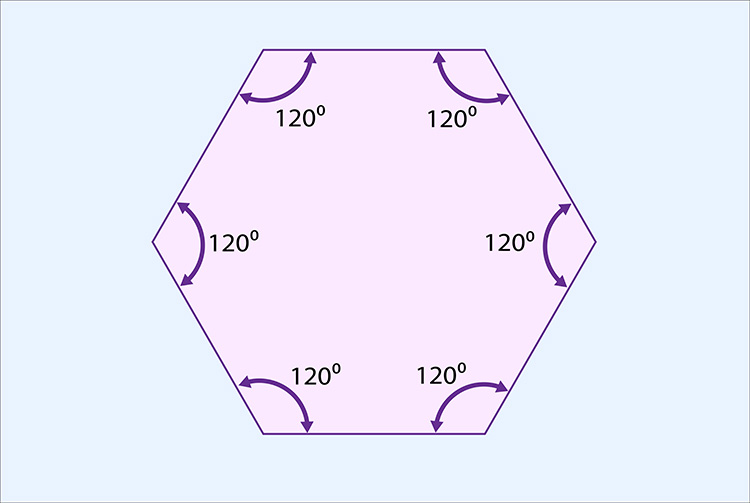# The total interior angles of a hexagon = 720°

Each time we add a side (triangle to square, square to pentagon, pentagon to hexagon) we add another 180°.

## Triangle to hexagon

 Triangle Square Pentagon Hexagon 180° + 180° + 180° + 180° = 720°

The total internal angles of a hexagon = 720°Now divide the total internal angle by the number of corners.

i.e. divide 720° by 6.

720^circ/6=120^circ

 1 2 3 4 5 6 120° + 120° + 120° + 120° + 120° + 120° = 720°